## Implement reflections with a local cubemap

This section of the guide shows you how to combine reflections based on local cubemaps with other types of reflections. For example, reflections rendered at runtime in your own custom shader. Reflections that are based on a local cubemap are a useful technique for rendering reflections on mobile devices. Unity version 5 and higher implements reflections based on local cubemaps like `Reflection Probes`.

Graphics developers have always tried to find computationally cheap methods to implement reflections.

In 1999, it became possible to use cubemaps with hardware acceleration. Cubemaps solved the problems of image distortions, viewpoint dependency and, computational inefficiency which previously related to spherical mapping techniques.

Cubemapping uses the six faces of a cube as the map shape. The environment is projected onto each side of a cube and stored as six square textures or unfolded into six regions of a single texture. By rendering the scene from a given position with six different camera orientations with a 90° view frustum representing each a cube face, generating the cubemap. Source images are sampled directly. No distortion is introduced by resampling into an intermediate environment map.

The following image shows an unfolded cube:The following image shows infinite reflections:To implement reflections based on cubemaps, evaluate the reflected vector R and use it to fetch the texel from the cubemap. In this example, `_Cubemap`, uses the available texture lookup function `texCUBE()`:

`float4 color = texCUBE(_Cubemap, R);`

The normal `N` and view vector `D` are passed to the fragment shader from the vertex shader. The fragment shader fetches the texture color from the cubemap, as shown in the following code:

```float3 R = reflect(D, N);
float4 color = texCUBE(_Cubemap, R);
```

Implementing reflections based on cubemaps can only reproduce reflections correctly from a distant environment where the cubemap position is not relevant. This simple and effective technique is mainly used in outdoor lighting, for example, to add reflections of the sky.

The following image shows incorrect reflections: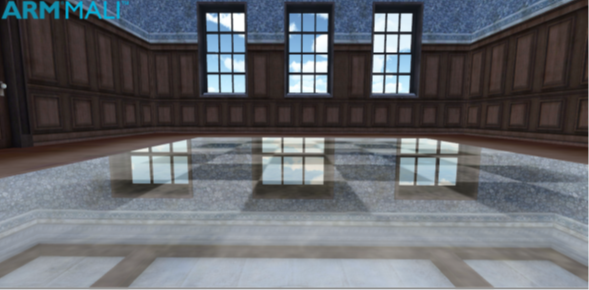If you use this infinite cubemaps in a local environment, it produces inaccurate reflections. The reflections are incorrect because in the following expression:

`float4 color = texCUBE(_Cubemap, R);`

There is no binding to the local geometry. For example, if you walk across a reflective floor looking at it from the same angle you always see the same reflection. The reflected vector is always the same and the expression always produces the same result. This is because the direction of the view vector does not change. In the real world, reflections depend on both viewing angle and viewing position.

#### Generate correct reflections with a local cubemap

A solution to the problem of incorrect reflections of local geometry involves binding to the local geometry in the procedure to calculate the reflection.

Note: This solution is described in GPU Gems: Programming Techniques, Tips, and Tricks for Real-time Graphics by Randima Fernando (Series Editor).

A bounding sphere is used as a proxy volume to delimit the scene that needs to be reflected. Instead of using the reflected vector R to fetch the texture from the cubemap a new vector R’ is used. To build the new vector, find the intersection point P in the bounding sphere of the ray, from the local point V in the direction of the reflected vector R. Create a new vector R’ from the center of the cubemap C, where the cubemap was generated, to the intersection point P.

The following image shows a local correction using a bounding sphere:Use this vector to fetch the texture from the cubemap:

```float3 R = reflect(D, N);
Find intersection point P
Find vector R’ = CP
float4 col = texCUBE(_Cubemap, R’);
```

This approach produces good results in the surfaces of objects with a near spherical shape but deforms reflections in plane reflective surfaces. Another problem with this method is that the algorithm that is used to calculate the intersection point with the bounding sphere involves solving a second-degree equation. This solution is relatively expensive.

In 2010 a developer proposed a better solution in a forum at Gamedev. The new approach replaces the previous bounding sphere with a box. This replacment solves the deformations and complexity problems of the previous method.

The following image shows a local correction using a bounding box:In 2012 Sebastien Lagarde used this approach to simulate more complex ambient specular lighting. This is done by using several cubemaps and an algorithm to evaluate the contribution of each cubemap and efficiently blend on the GPU.

The following table shows the differences between infinite and local cubemaps:

 Infinite Cubemaps Local Cubemaps Mainly used outdoors to represent the lighting from a distant environment Cubemap position is not relevant. Represents the lighting from a finite local environment Cubemap position is relevant. The lighting from local cubemaps is only correct at the location where the cubemap was created. Local correction must be applied to adapt the intrinsic infinite nature of cubemaps to local environment.

The following image is from the same scene as the one shown in the introductory paragraph. However, this version of the scene contains the correct reflections generated with local cubemaps: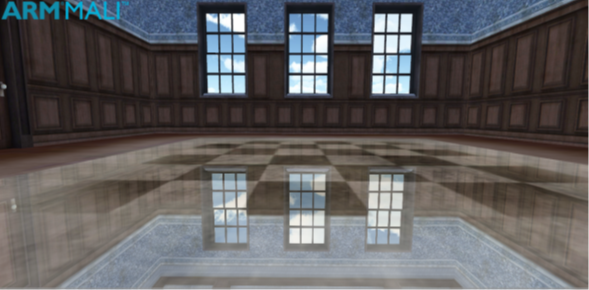This section shows shader implementation of reflections using local cubemaps in Unity.

The vertex shader calculates three magnitudes that are passed to the fragment shader as interpolated values:

• The vertex position
• The view direction
• The normal

These values are in world coordinates.

The following code shows a shader implementation of reflections using local cubemaps, for Unity:

```vertexOutput vert(vertexInput input)
{
vertexOutput output;
output.tex = input.texcoord;
// Transform vertex coordinates from local to world.
float4 vertexWorld = mul(_Object2World, input.vertex);
// Transform normal to world coordinates.
float4 normalWorld = mul(float4(input.normal,0.0), _World2Object);
// Final vertex output position. output.pos = mul(UNITY_MATRIX_MVP, input.vertex);
// ----------- Local correction ------------
output.vertexInWorld = vertexWorld.xyz;
output.viewDirInWorld = vertexWorld.xyz - _WorldSpaceCameraPos;
output.normalInWorld = normalWorld.xyz;
return output;
}
```

The intersection point in the volume box and the reflected vector are computed in the fragment shader. Build a new local corrected reflection vector and use it to fetch the reflection texture from the local cubemap. Then combine the texture and reflection to produce the output color:

```float4 frag(vertexOutput input) : COLOR
{
float4 reflColor = float4(1, 1, 0, 0);
// Find reflected vector in WS.
float3 viewDirWS = normalize(input.viewDirInWorld);
float3 normalWS = normalize(input.normalInWorld);
float3 reflDirWS = reflect(viewDirWS, normalWS);
// Working in World Coordinate System.
float3 localPosWS = input.vertexInWorld;
float3 intersectMaxPointPlanes = (_BBoxMax - localPosWS) / reflDirWS;
float3 intersectMinPointPlanes = (_BBoxMin - localPosWS) / reflDirWS;
// Looking only for intersections in the forward direction of the ray.
float3 largestParams = max(intersectMaxPointPlanes, intersectMinPointPlanes);
// Smallest value of the ray parameters gives us the intersection.
float distToIntersect = min(min(largestParams.x, largestParams.y), largestParams.z);
// Find the position of the intersection point.
float3 intersectPositionWS = localPosWS + reflDirWS * distToIntersect;
// Get local corrected reflection vector.
float3 localCorrReflDirWS = intersectPositionWS - _EnviCubeMapPos;
// Lookup the environment reflection texture with the right vector.
reflColor = texCUBE(_Cube, localCorrReflDirWS);
// Lookup the texture color.
float4 texColor = tex2D(_MainTex, input.tex);
return _AmbientColor + texColor * _ReflAmount * reflColor;
}
```

In the preceding fragment shader code, the magnitudes `_BBoxMax` and `_BBoxMin` are the maximum and minimum points of the bounding volume. The variable `_EnviCubeMapPos` is the position where the cubemap was created. Pass these values to the shader from the following script:

```[ExecuteInEditMode]
public class InfoToReflMaterial : MonoBehaviour
{
// The proxy volume used for local reflection calculations.
public GameObject boundingBox;
void Start()
{
Vector3 bboxLength = boundingBox.transform.localScale;
Vector3 centerBBox = boundingBox.transform.position;
// Min and max BBox points in world coordinates
Vector3 BMin = centerBBox - bboxLength/2;
Vector3 BMax = centerBBox + bboxLength/2;
// Pass the values to the material.
gameObject.renderer.sharedMaterial.SetVector("_BBoxMin", BMin);
gameObject.renderer.sharedMaterial.SetVector("_BBoxMax", BMax);
gameObject.renderer.sharedMaterial.SetVector("_EnviCubeMapPos", centerBBox);
}
}
```

Pass the values for `_AmbientColor`, `_ReflAmount`, the main texture, and cubemap texture to the shader as uniforms from the properties block:

```Shader "Custom/ctReflLocalCubemap"
{
Properties
{
_MainTex ("Base (RGB)", 2D) = "white" { }
_Cube("Reflection Map", Cube) = "" {}
_AmbientColor("Ambient Color", Color) = (1, 1, 1, 1)
_ReflAmount("Reflection Amount", Float) = 0.5
}
{
Pass
{
CGPROGRAM
#pragma glsl
#pragma vertex vert
#pragma fragment frag
#include "UnityCG.cginc"
// User-specified uniforms
uniform sampler2D _MainTex;
uniform samplerCUBE _Cube;
uniform float4 _AmbientColor;
uniform float _ReflAmount;
uniform float _ToggleLocalCorrection;
// ----Passed from script InfoRoReflmaterial.cs --------
uniform float3 _BBoxMin;
uniform float3 _BBoxMax;
uniform float3 _EnviCubeMapPos;
struct vertexInput
{
float4 vertex : POSITION;
float3 normal : NORMAL;
float4 texcoord : TEXCOORD0;
};
struct vertexOutput
{
float4 pos : SV_POSITION;
float4 tex : TEXCOORD0;
float3 vertexInWorld : TEXCOORD1;
float3 viewDirInWorld : TEXCOORD2;
float3 normalInWorld : TEXCOORD3;
};
ENDCG
}
}
}
```

The algorithm that is used to calculate the intersection point in the bounding volume is based on the use of the parametric representation of the reflected ray from the local position or fragment. For a description of the ray-box intersection algorithm, see Ray-box intersection algorithm

#### Filtering cubemaps

This section shows you to filter cubemaps after you have implemented reflections using local cubemaps using the CubeMapGen tool.

One of the advantages of implementing reflections using local cubemaps is the fact that the cubemap is static. This means that the cubemap is generated during development, rather than at runtime. Generating the cubemap during development provides an opportunity to apply any filtering to the cubemap images to achieve an effect.

To export cubemap images from Unity to CubeMapGen, you must save each cubemap image separately. It is best to use Legacy Cubemaps in Unity for this procedure, to make it easy to transfer individual images between the two tools.  For the source code of a tool that saves the images, see Source code to generate cubemaps. This tool can create a cubemap and can optionally save each cubemap image separately.

Note: CubeMapGen is a legacy tool by AMD that applies filtering to cubemaps.

You must place the script for this tool in a folder called Editor in the Asset directory.

To use the cubemap editor tool:

1. Create the cubemap.
2. Launch the Bake CubeMap tool from the GameObject menu.
3. Provide the cubemap and the camera render position.
4. Optionally save individual images if you plan to apply filtering to the cubemap.

The following screenshot shows the Bake CubeMap tool interface: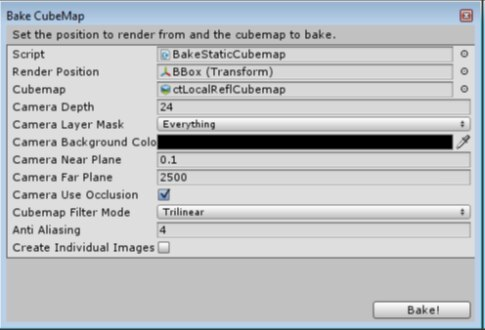You can load each of the images for the cubemap separately with CubeMapGen.

To load a face, select one from the Select Cube Face drop-down menu and then click Load Cubemap Face. When all faces have been loaded, rotate the cubemap and check that it is correct.

CubeMapGen has several filtering options in the Filter Type drop down menu. Select the filter settings that you require and click Filter cubemap to apply the filter. The filtering can take several minutes depending on the size of the cubemap.

Note: There is no undo option, so save the cubemap as a single image before applying any filtering. If the result of the filtering is not what you expect it to be, reload the cubemap and adjust the parameters.

Use the following procedure for importing cubemap images into CubeMapGen:

1. Make sure you have saved individual images by checking the Create Individual Images box when you baked the cubemap in Unity
2. Launch the CubeMapGen tool and load cubemap images following the relations that are shown in the following table:
 AMD CubeMapGen Unity X+ -X X- +X Y+ +Y Y- -Y Z+ +Z Z- -Z
3. Save the cubemap as a single dds or cube cross image.
Note: There is no undo, therefore save the cubemap as a single image before filtering, enabling you to reload the cubemap if you are experimenting with filters.
4. Apply filters to the cubemap as required until the results are satisfactory.
5. Save the cubemap as individual images.

The following screenshot shows the input image in CubeMapGen after loading the six cubemap images: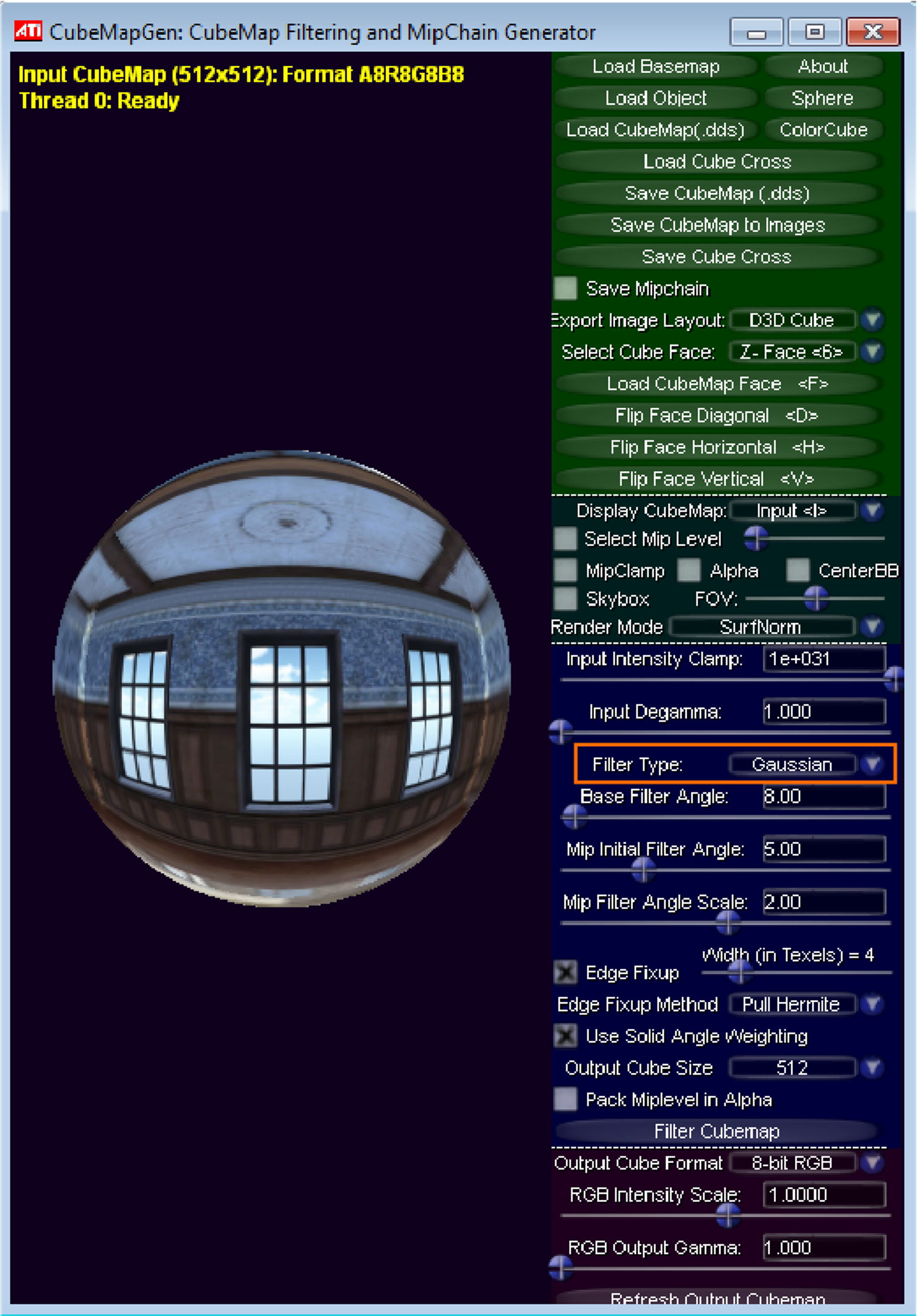The following screenshot shows the output result of CubeMapGen after applying a Gaussian filtering to achieve a frosty effect: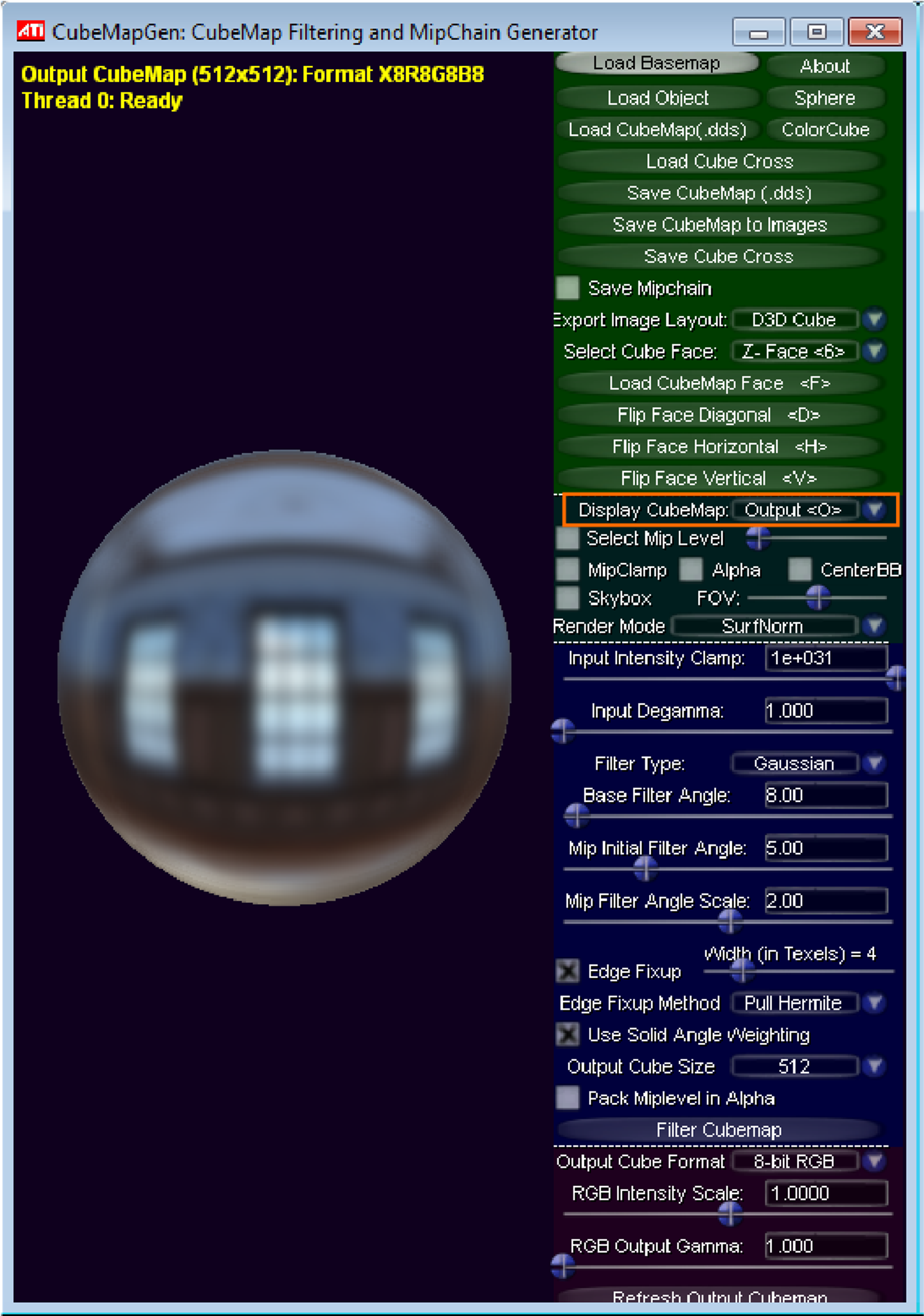The following table shows the filter parameters used with the Gaussian filter to achieve the frosty effect:

 Filter settings Value Type Gaussian Base Filter Angle 8 Mip Initial Filter Angle 5 Mip Filter Angle Scale 2.0 Edge Fixup Checked Edge Fixup Width 4

The following image shows a reflection that is generated with a cubemap with a frosty effect: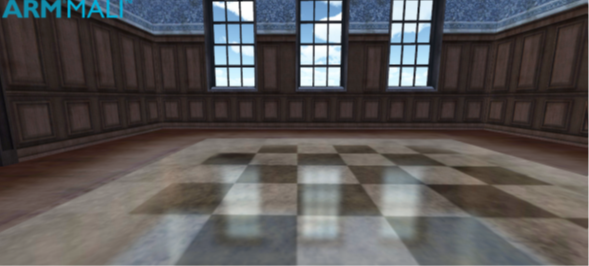The following image summarizes the preceding workflow that is used to apply filtering to Unity cubemaps with the CubeMapGen tool:#### Ray-box intersection algorithm

This section describes the ray-box intersection algorithm. The ray-box intersection algorithm was mentioned in Shader implementations.

Equation of a line:

`y = mx + b`

The vector form of this equation is:

`r = O + t*D`

Where

• `O` is the origin point
• `D` is the direction vector
• `t` is the parameter

The following image shows an axis aligned bounding box:The min and max points A and B define an axis-aligned bounding box `AABB`.

`AABB` defines a set of lines parallel to coordinate axis. The following equation defines each component of the line:

```x = Ax; y = Ay; z = Az
x = Bx; y = By; z = Bz
```

To find where a ray intersects one of those lines, make both equations equal. For example:

```Ox + tx*Dx = Ax
```

You can write the solution as:

`tAx = (Ax – Ox) / Dx`

Obtain the solution for all components of both intersection points in the same manner:

```tAx = (Ax – Ox) / Dx
tAy = (Ay – Oy) / Dy
tAz = (Az – Oz) / Dz
tBx = (Bx – Ox) / Dx
tBy = (By – Oy) / Dy
tBz = (Bz – Oz) / Dz
```

In vector form, they are:

```tA = (A – O) / D
tB = (B – O) / D
```

The solution finds where the line intersects the planes that are defined by the faces of the cube, but it does not guarantee that the intersections lie on the cube.

The following image shows a 2D representation of ray-box intersection:To find the solution that intersects with the box, you require the larger value of t for the intersection at the `min` plane:

```tmin = (tAx > tAy) ? tAx: tAy
tmin = (tmin > tAz) ? tmin: tAz
```

You require the smaller value of t for the intersection at the max plane:

```tmax = (tAx < tAy) ? tAx: tAy
tmax = (tmin < tAz) ? tmin: tAz
```

Note: The ray does not always intersect the box.

The following image shows a ray-box with no intersection:If you guarantee that the `BBox` encloses the reflective surface, that is, the origin of the reflected ray is inside the `BBox`. This means that there are always two intersections with the box, and the handling of different cases is simplified.

The following image shows a ray-box intersection in `BBox`:#### Source code for editor script to generate cubemaps

• This section provides the source code for the editor script to generate cubemaps:
```/*
* This confidential and proprietary software may be used only as
* authorised by a licensing agreement from Arm Limited* (C) COPYRIGHT 2014 Arm Limited* ALL
RIGHTS RESERVED
* The entire notice above must be reproduced on all authorised
* copies and copies may only be made to the extent permitted
* by a licensing agreement from Arm Limited.
*/
using UnityEngine;
using UnityEditor;
using System.IO;
/**
* This script must be placed in the Editor folder.
* The script renders the scene into a cubemap and optionally
* saves each cubemap image individually.
* The script is available in the Editor mode from the
* Game Object menu as "Bake Cubemap" option.
* Be sure the camera far plane is enough to render the scene.
*/
public class BakeStaticCubemap : ScriptableWizard
{
public Transform renderPosition;
public Cubemap cubemap;
// Camera settings.
public Color cameraBackgroundColor;
public float cameraNearPlane = 0.1f;
public float cameraFarPlane = 2500.0f;
public bool cameraUseOcclusion = true;
// Cubemap settings.
public FilterMode cubemapFilterMode = FilterMode.Trilinear;
// Quality settings.
public int antiAliasing = 4;
public bool createIndividualImages = false;
// The folder where individual cubemap images will be saved
static string imageDirectory = "Assets/CubemapImages";
static string[] cubemapImage = new string[]{"front+Z", "right+X", "back-Z", 	"left-X", "top+Y", "bottom-Y"};
static Vector3[] eulerAngles = new Vector3[]{new Vector3(0.0f,0.0f,0.0f), new 	Vector3(0.0f,-90.0f,0.0f), new Vector3(0.0f,180.0f,0.0f), new 	Vector3(0.0f,90.0f,0.0f), new Vector3(-90.0f,0.0f,0.0f), new 	Vector3(90.0f,0.0f,0.0f)};

void OnWizardUpdate()
{
helpString = "Set the position to render from and the cubemap to bake.";
if(renderPosition != null && cubemap != null)
{
isValid = true;
}
else
{
isValid = false;
}
}

void OnWizardCreate ()
{
// Create temporary camera for rendering.
GameObject go = new GameObject( "CubemapCam", typeof(Camera) );
// Camera settings.
go.camera.backgroundColor = cameraBackgroundColor;
go.camera.nearClipPlane = cameraNearPlane;
go.camera.farClipPlane = cameraFarPlane;
go.camera.useOcclusionCulling = cameraUseOcclusion;
// Cubemap settings
cubemap.filterMode = cubemapFilterMode;
// Set antialiasing
QualitySettings.antiAliasing = antiAliasing;
// Place the camera on the render position.
go.transform.position = renderPosition.position;
go.transform.rotation = Quaternion.identity;
// Bake the cubemap
go.camera.RenderToCubemap(cubemap);
// Rendering individual images
if(createIndividualImages)
{
if (!Directory.Exists(imageDirectory))
{
Directory.CreateDirectory(imageDirectory);
}
RenderIndividualCubemapImages(go);
}
// Destroy the camera after rendering.
DestroyImmediate(go);
}
void RenderIndividualCubemapImages(GameObject go)
{
go.camera.backgroundColor = Color.black;
go.camera.clearFlags = CameraClearFlags.Skybox;
go.camera.fieldOfView = 90;
go.camera.aspect = 1.0f;
go.transform.rotation = Quaternion.identity;
//Render individual images
for (int camOrientation = 0; camOrientation < eulerAngles.Length ; camOrientation++)
{
string imageName = Path.Combine(imageDirectory, cubemap.name + "_" + cubemapImage[camOrientation] + ".png");
go.camera.transform.eulerAngles = eulerAngles[camOrientation];
RenderTexture renderTex = new RenderTexture(cubemap.height, cubemap.height, cameraDepth);
go.camera.targetTexture = renderTex;
go.camera.Render();
RenderTexture.active = renderTex;
Texture2D img = new Texture2D(cubemap.height, cubemap.height, TextureFormat.RGB24, false);
img.ReadPixels(new Rect(0, 0, cubemap.height, cubemap.height), 0, 0);
RenderTexture.active = null;
GameObject.DestroyImmediate(renderTex);
byte[] imgBytes = img.EncodeToPNG();
File.WriteAllBytes(imageName, imgBytes);
AssetDatabase.ImportAsset(imageName, ImportAssetOptions.ForceUpdate);
}
AssetDatabase.Refresh();
}